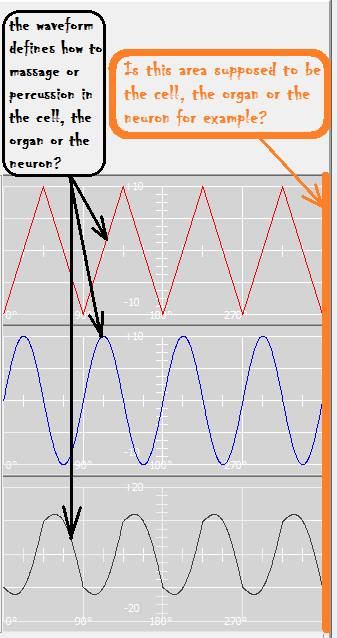# Can someone explain this waveform area to me?No, nothing so esoteric as that. What those lines are is a visual representation of what the output voltage is doing over a period of time. What you're looking at is called the waveform.

The top graph there shows the how the voltage will change at Out1. That's a representation, or graph, of a triangle wave. You can see the white horizontal lines with the little "tick" marks on them in all three graphs. That line represents zero volts. And see that none of the waveforms pass through zero volts in step with each other. That's because Out1 and Out2 (the second graph) are out of phase. But if you look closely, you see that each of the first two graphs pass through zero every other "tick" mark. That means they are running at the same frequency.

The second graph is what the voltage at Out1 looks like and that waveform is a Sine wave. The sine wave is short for the sinusoidal wave which is a math term. If you look at the white line in the middle that also has the "tick" marks, you'll see the +10 and -10. Those are the voltage scale. So in both of the first two graphs, the waveform's peak at plus and minus 10 Volts. The degree marks at the bottom represent the time during one cycle, where one cycle is 360 degrees. In the graphs you've posted, it looks like you must be using a wave cycle multiplier of 4. If you had a wave cycle multiplier of 1, there would be fewer ups and downs. Try fooling with the settings and it will change. You can also change the voltage of each waveform over at the top left of the Settings page under "Amplitude".

The bottom graph is what you get at the output of the Boost. It's the combination of the two waves. Also note that the voltage scale on the bottom graph is double the first two, because the boost can double the voltage by combining them.